# Excel如何取得股票代碼清單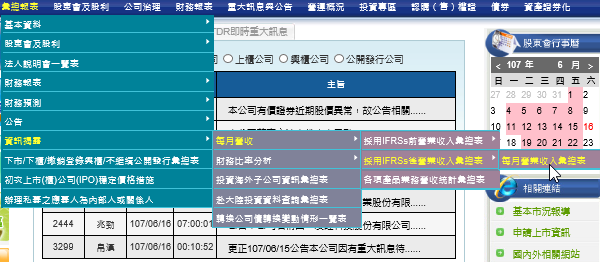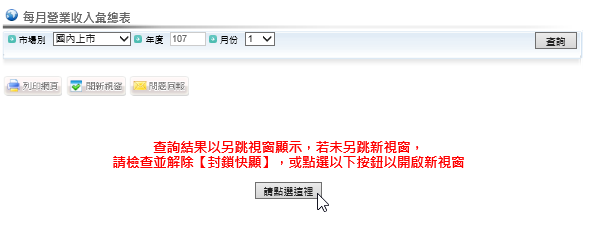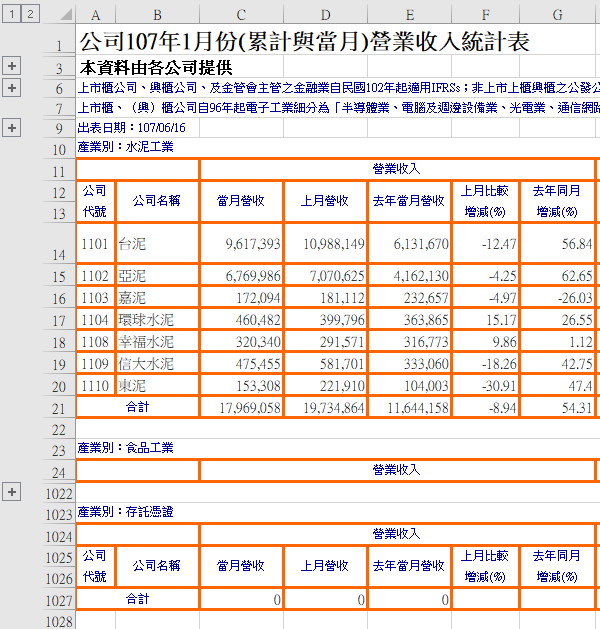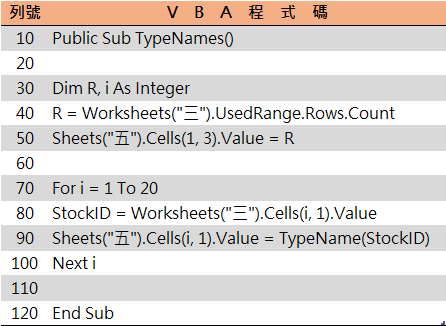R = Worksheets(“三”).UsedRange.Rows.Count

Sheets(“五”).Cells(1, 3).Value = R

StockID = Worksheets(“三”).Cells(i, 1).Value

Sheets(“五”).Cells(i, 1).Value = TypeName(StockID)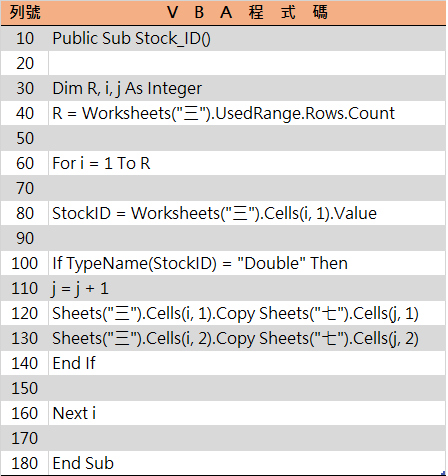R = Worksheets(“三”).UsedRange.Rows.Count

For i = 1 To R

StockID = Worksheets(“三”).Cells(i, 1).Value

If TypeName(StockID) = “Double” Then

j = j + 1

Sheets(“三”).Cells(i, 1).Copy Sheets(“七”).Cells(j, 1)

Sheets(“三”).Cells(i, 2).Copy Sheets(“七”).Cells(j, 2)

End If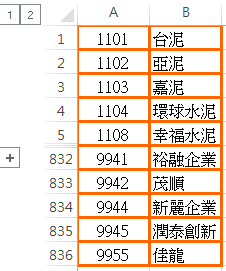VBA網路爬蟲